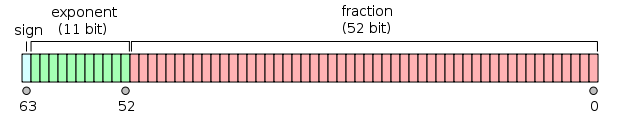# 说说 JavaScript 中的数字

April 20, 2019

JavaScript 中的数字（number 类型）占据 64 位，为 IEEE 754 规范的双精度浮点数，其二进制的表示形式如下：## 规约形式的浮点数(normal number)

((-1) ** sign) * 1.fraction * (2 ** (exponent - 1023))

## 非规约形式的浮点数(subnormal number)

((-1) ** sign) * 0.fraction * 2 ** -1022

## 关于整数

(-2 ** 53 + 1) ~ (2 ** 53 -1)

## 查看数字的二进制表示

function getNumberBits(n) {
const f64a = new Float64Array([n])
const v = new DataView(f64a.buffer)
let b = ''
for (let i = 7; i >= 0; i--) {
b += v
.getUint8(i)
.toString(2)
}
return {
bits: b,
sign: b,
exponent: b.slice(1, 1 + 11),
fraction: b.slice(1 + 11),
}
}

## 未来

BigInt 是一个新增的基本类型（Primitive），目前已经进入 Stage 3，有了 BigInt，我们就可以精确的表达更大的整数了。

# JavaScript
© 2015 - 2022 zhuscat
Hosted on Vercel